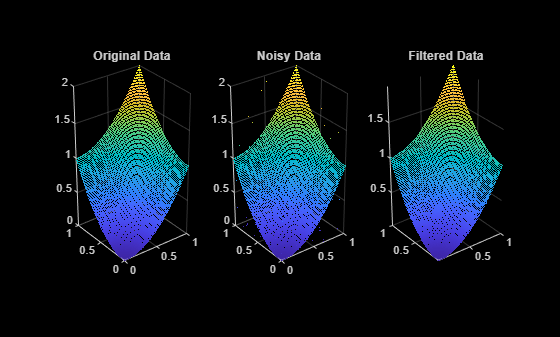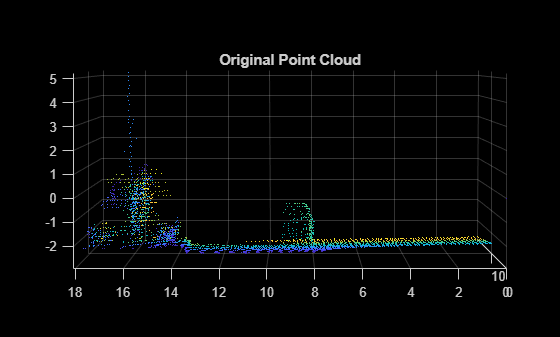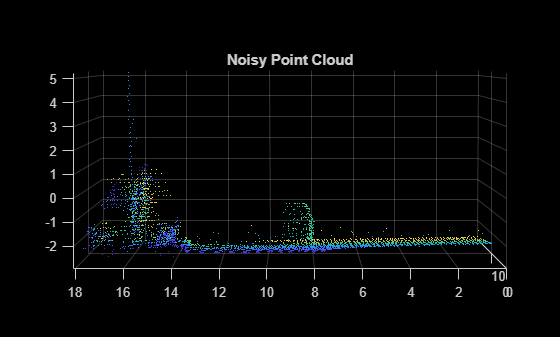pcmedian

Median filtering 3-D point cloud data

Description

example

ptCloudOut = pcmedian(ptCloudIn) performs median filtering of 3-D point cloud data. The function filters each channel of the point cloud individually. The output is a filtered point cloud. Each output location property value is the median of neighborhood around the corresponding input location property value. The pcmedian function doesn't pad zeros on the edges. Rather, it operates only on the available neighborhood values.

If the input point cloud is an organized point cloud, the pcmedian function uses N-by-N neighborhood method. If the point cloud is unorganized, the function uses radial neighborhood method.

example

ptCloudOut = pcmedian(___,Name,Value) specifies options using one or more name-value pair arguments. For example, 'FilterSize',3 sets the size of the median filter for organized point clouds to 3.

Examples

collapse all

Use the median filter to remove noise from a point cloud. First, add random noise to a point cloud. Then, use the pcmedian function to filter the noise.

Create a point cloud.

gv = 0:0.01:1;
[X,Y] = meshgrid(gv,gv);
Z = X.^2 + Y.^2;
ptCloud = pointCloud(cat(3,X,Y,Z));

Add random noise along the z-axis.

temp = ptCloud.Location;
count = numel(temp(:,:,3));
temp((2*count) + randperm(count,100)) = rand(1,100);
temp(count + randperm(count,100)) = rand(1,100);
temp(randperm(count,100)) = rand(1,100);
ptCloudA = pointCloud(temp);

Apply the median filter and display the three point clouds (original, noisy, and filtered).

ptCloudB = pcmedian(ptCloudA);

subplot(1,3,1)
pcshow(ptCloud)
title('Original Data')
subplot(1,3,2)
pcshow(ptCloudA)
title('Noisy Data')
subplot(1,3,3)
pcshow(ptCloudB)
title('Filtered Data')Load point cloud data into the workspace.

roi = [0 20 0 20 -5 15];
indices = findPointsInROI(ptCloud,roi);
ptCloud = select(ptCloud,indices);
ptCloud = pcdownsample(ptCloud,'gridAverage',0.2);

Display the point cloud data. Each point is color-coded based on its x-coordinate.

figure
pcshow(ptCloud.Location,ptCloud.Location(:,1))
view(-90,2)
title('Original Point Cloud')Add noise along z-channel in the interval (a,b). Values of a and b are chosen to make the noise appear close to the ground.

temp = ptCloud.Location;
count = numel(temp(:,3));
a = -2.5;
b = -2;
temp((2*count)+randperm(count,200)) = a+(b-a).*rand(1,200);
ptCloudA = pointCloud(temp);

Display the noisy point cloud. Each point is color-coded based on its x-coordinate.

figure
pcshow(ptCloudA.Location,ptCloudA.Location(:,1))
view(-90,2)
title('Noisy Point Cloud')Apply median filter on the point cloud.

Display the filtered point cloud. Each point is color-coded based on its x-coordinate.

figure
pcshow(ptCloudB.Location, ptCloudB.Location(:,1))
view(-90,2)
title('Filtered Point Cloud')Input Arguments

collapse all

Point cloud, specified as a pointCloud object with at least one valid point. If the input point cloud is organized, the size of the point cloud must be at least 3-by-3-by-3.

Name-Value Arguments

Specify optional comma-separated pairs of Name,Value arguments. Name is the argument name and Value is the corresponding value. Name must appear inside quotes. You can specify several name and value pair arguments in any order as Name1,Value1,...,NameN,ValueN.

Example: 'FilterSize',3 specifies a median filter size of 3.

Point cloud dimensions of interest, specified as a vector of integers in the range [1 3]. The values 1, 2, and 3 correspond to the x-, y-, and z-axis respectively. You must specify dimensions in ascending order.

Data Types: single | double | int8 | int16 | int32 | int64 | uint8 | uint16 | uint32 | uint64

Size of the median filter for an organized point cloud, specified as an odd integer in the range [3, N]. N is the smallest of channel dimensions in the point cloud.

Data Types: single | double | int8 | int16 | int32 | int64 | uint8 | uint16 | uint32 | uint64

Radius of the neighborhood for unorganized point cloud, specified as a positive scalar. The computation time increases when there are a lot of points inside the specified radius. So, large radius values for dense point clouds can cause high computation time and impact performance.

Data Types: single | double

Output Arguments

collapse all

Filtered point cloud, returned as a pointCloud object.

Extended Capabilities

Objects

Introduced in R2020b

Lidar Toolbox Documentation